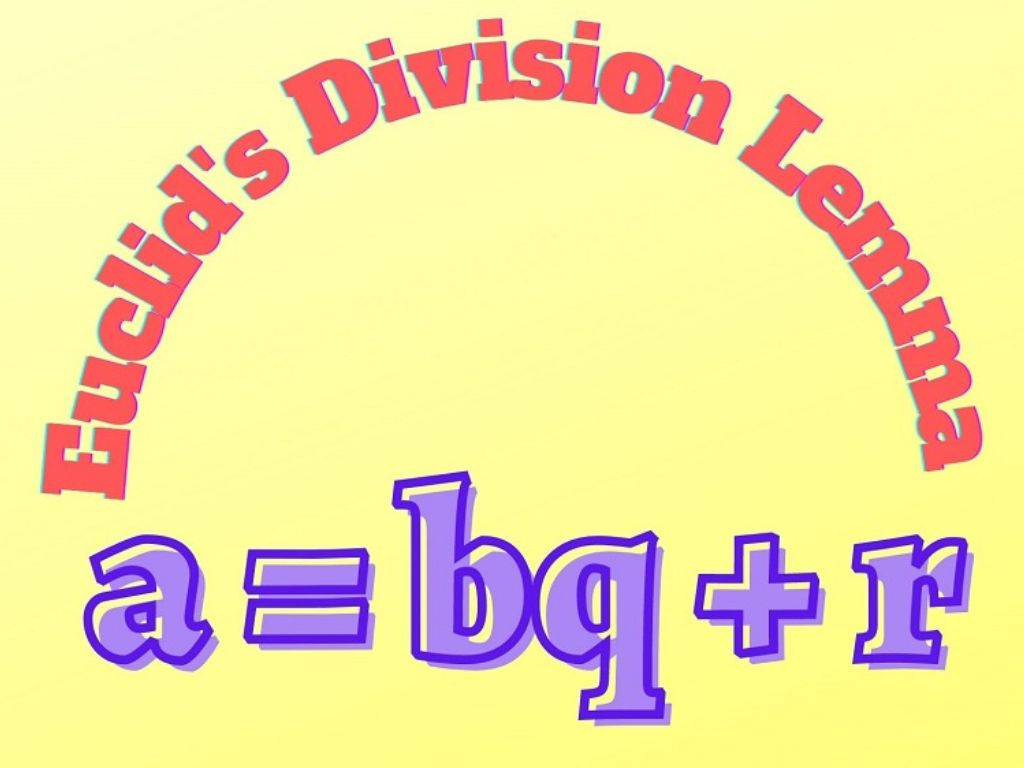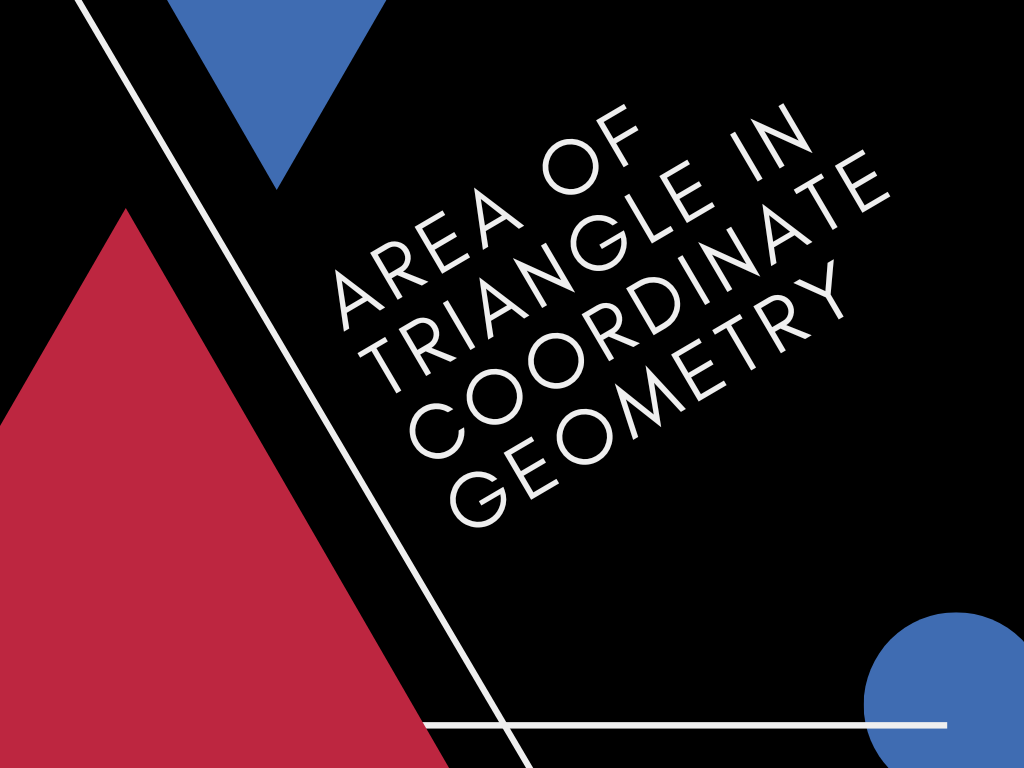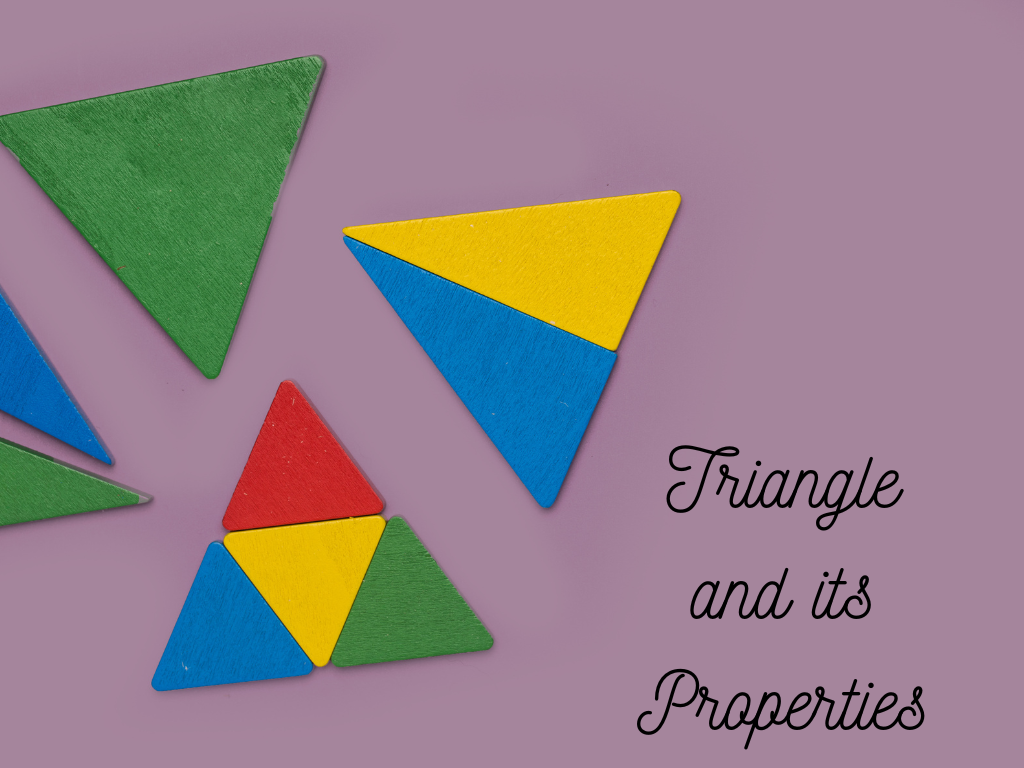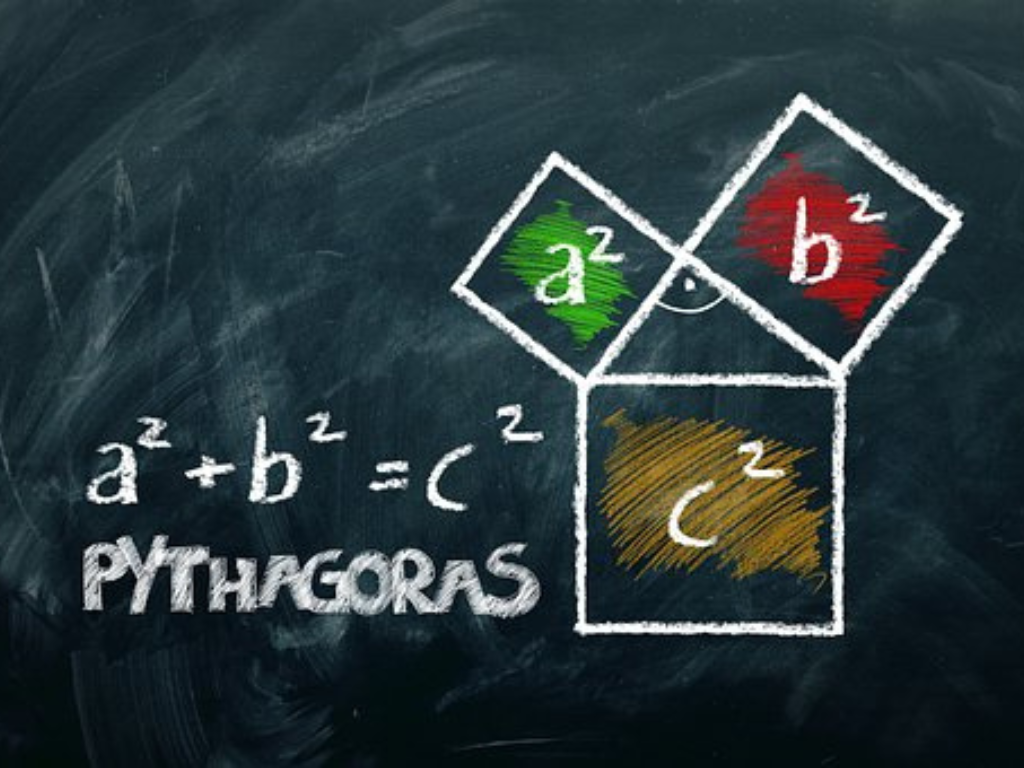# April 2021## The Fundamental Theorem of Arithmetic Class 10th

Introduction The Fundamental Theorem of Arithmetic is related to Number System. We have already studied prime numbers and composite numbers. The numbers which are divisible by 1 or the number itself are called Prime Numbers and the numbers which are divisible by other numbers also are called Composite Numbers. The factors of a prime number …## Euclid’s Division Lemma Class 10th

Introduction Euclid’s Division Lemma is generally an algorithm that is derived by Greek Mathematician Euclid. This lemma is based on the Division of Real Numbers. We have already studied the division algorithm, in which if we divide a number by another number we get two numbers a quotient and a remainder (sometimes the remainder may …## Area of Triangle in Coordinate Geometry Class 10th

Introduction Before finding the Area of a Triangle in Coordinate Geometry, We know the area of a triangle if its Base and Height are given Area of triangle = ½⨯Base⨯Height If all three sides of a triangle are given then the area of the triangle by Heron’s formula Area of triangle = √s(s-a)(s-b)(s-c)                     Where, s …## Arithmetic Progression Class 10th

Introduction In our surroundings, we must have observed that many things follow certain patterns or designs such as tiles mounted on the floor at home, stripes of a wheel, Stairs, shapes on watermelon, etc. this certain pattern or design is called a sequence. In some sequence, patterns remain the same but they come repeatedly and …## Triangle and its Properties Class 10th

Definition A Closed figure made of three line segments is called a Triangle. It has three sides, three angles, and three vertices. Here, Triangle ABC and its Properties are shown below. Three sides – AB, BC, CA Three angles – ∠A, ∠B, ∠C Three vertices – A, B, C Classification of Triangles – ➤ Based on …## Pythagoras Theorem (Boudhayana Theorem) Class 10th

Introduction The Pythagoras theorem is given by the Greek mathematician Pythagoras. Before Pythagoras, this theorem was discovered by Indian mathematician Boudhayana so this theorem is also known as Boudhayana Theorem. This theorem is also called Pythagorean Theorem. Statement – According to this theorem, In a right-angled triangle, the square of the hypotenuse side is equal …